# SAT II Math I : Perimeter

## Example Questions

### Example Question #23 : 2 Dimensional Geometry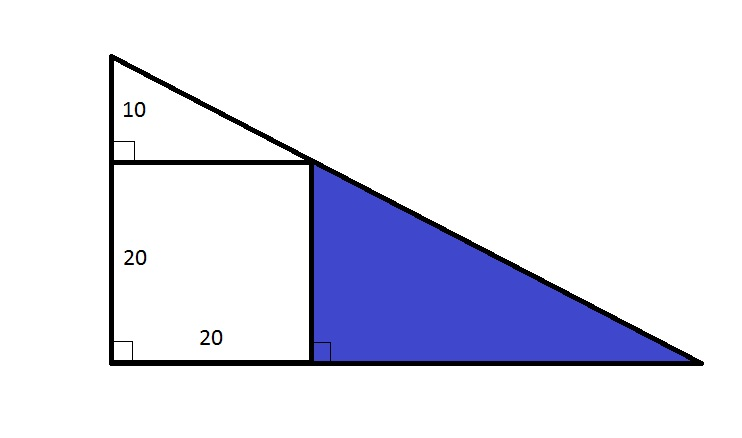Note: Figure NOT drawn to scale.

Refer to the figure above, which shows a square inscribed inside a large triangle. What is the difference between the perimeter of the white trapezoid and the blue triangle?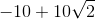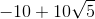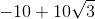Explanation:

One side of the white trapezoid is the hypotenuse of the small top triangle, which has legs 10 and 20. Therefore, the length of this side can be determined using the Pythagorean Theorem: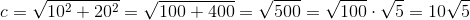The trapezoid has perimeter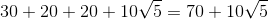.

The small top triangle has legs 10 and 20 and hypotenuse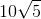, and, therefore, perimeter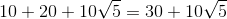. The blue triangle, which is similar as a result of the parallelism of the opposite sides of the square, has short leg 20. Since the perimeter of two similar triangles is directly proportional to a side, we can set up and solve the proportion statement to find the perimeter of the blue triangle: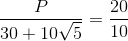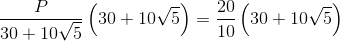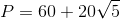The difference between the perimeters is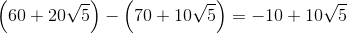### Example Question #1 : Perimeter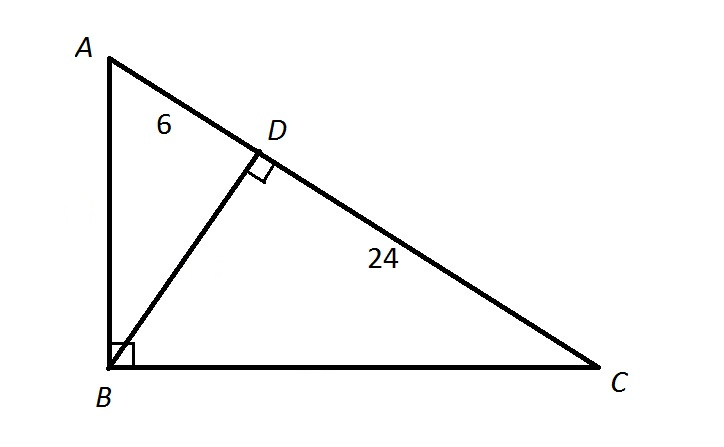Note: Figure NOT drawn to scale.

Refer to the above diagram. Give the ratio of the perimeter of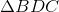to that of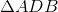.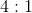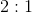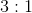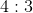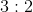Explanation:

The altitude of a right triangle from the vertex of its right triangle to its hypotenuse divides it into two similar triangles.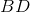, as the length of the altitude corresponding to the hypotenuse, is the geometric mean of the lengths of the parts of the hypotenuse it forms; that is, it is the square root of the product of the two: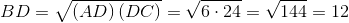.

The ratio of the smaller side ofto that ofis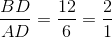or 2:1, making this the similarity ratio. Theratio of the perimeters is always equal to the similarity ratio, so 2:1 is also the ratio of the perimeter ofto that of.

### Example Question #25 : 2 Dimensional Geometry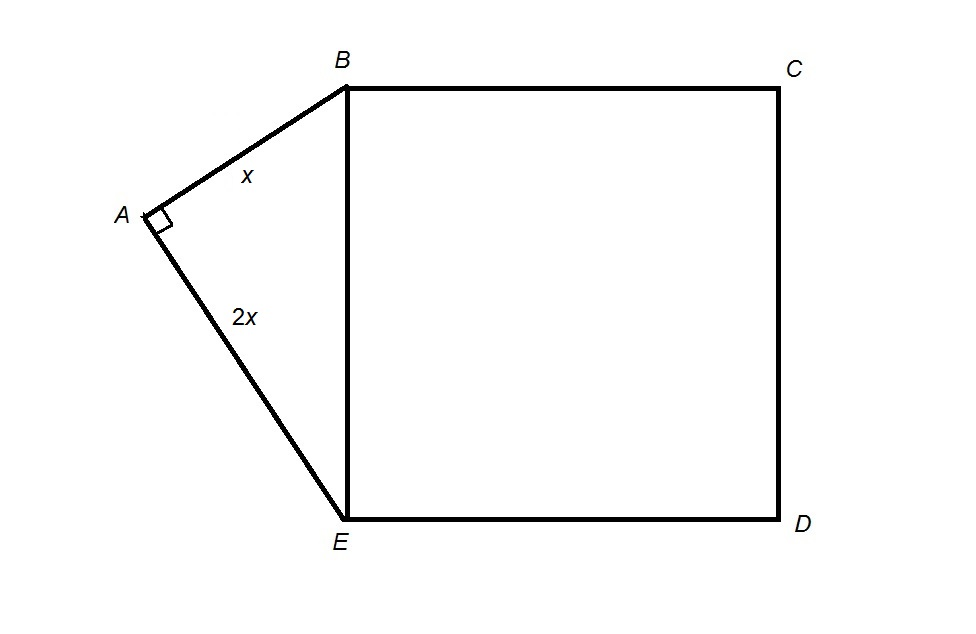Refer to the above figure. Quadrilateral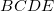is a square. Give the perimeter of Polygon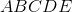in terms of.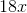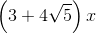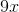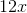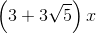Explanation: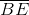is both one side of Squareand the hypotenuse of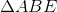;  its hypotenuse can be calculated from the lengths of the legs using the Pythagorean Theorem: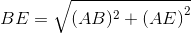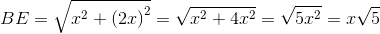Therefore,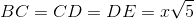.

The perimeter of Polygonis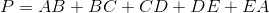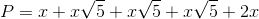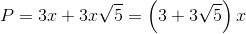### Example Question #26 : 2 Dimensional Geometry

The area of a rectangle is 16.  Assuming the length and width are integers, which of the answers is NOT a possible perimeter?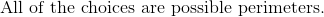Explanation:

The area of a rectangle is length times width.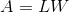Determine all the integer combinations that will multiply to an area of 16.  The numbers can represent length or width interchangably.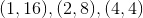Write the perimeter formula for a rectangle.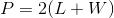Substitute all the following combinations to determine the perimeters.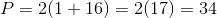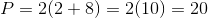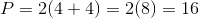The maximum allowable perimeter is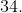The perimeter that is not possible is.

### Example Question #1 : Perimeter

If a side of a square has a length of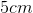, what is the perimeter?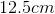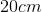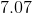Explanation:

Step 1: Define Perimeter...

The perimeter of any shape is the sum of all the sides of that figure..

Step 2: Find how many sides a square has...

A square hassides.

Step 3: Calculate the perimeter...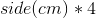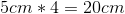### Example Question #28 : 2 Dimensional Geometry

What is the perimeter of a rectangle if the area is 60, and one of the side length is 15?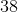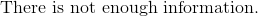Explanation:

Write the formula for the area of the rectangle.  We will need the length of the other side of the rectangle.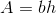Let the known side length be the base.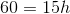Divide by 15 on both sides to get the height.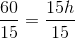There are two bases and two heights in a rectangle.

The perimeter is: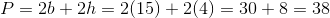The answer is:### Example Question #29 : 2 Dimensional Geometry

Find the perimeter of an equilateral triangle with a length of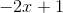.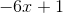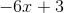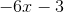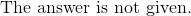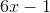Explanation:

An equilateral triangle has three congruent side lengths.

This means that the perimeter has to be triple the side length.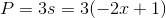Distribute the three with both terms of the binomial.

The answer is: